# 【机器学习详解】SVM解回归问题

CSDN

## 1.方法分析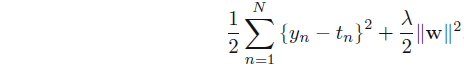ϵinsensitive$\epsilon-insensitive$ 误差函数定义为，如果预测值yn$y_n$与真实值tn$t_n$的差值小于阈值ϵ$\epsilon$将不对此样本点做惩罚，若超出阈值，惩罚量为|yntn|ϵ$|y_n-t_n|-\epsilon$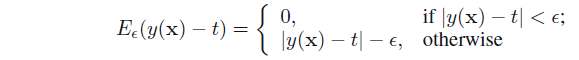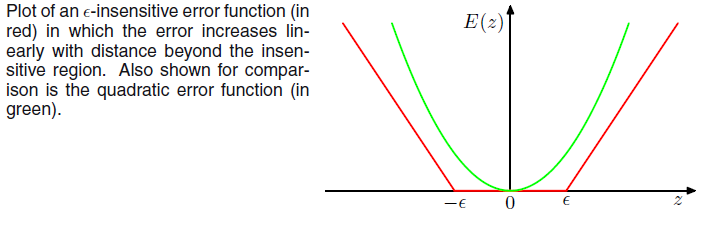## 2.目标函数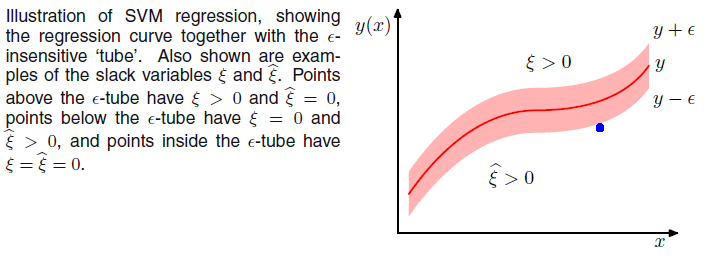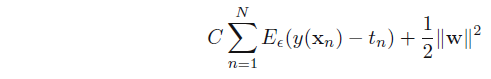tn$t_n$位于管道上方时，ξn>0$\xi_n>0$，有tny(xn)ξnϵ$t_n-y(x_n)-\xi_n\leq\epsilon$
tn$t_n$位于管道下方时，ξn^>0$\hat{\xi_n}>0$，有y(xn)tnξ^nϵ$y(x_n)-t_n-\hat{\xi}_n\leq\epsilon$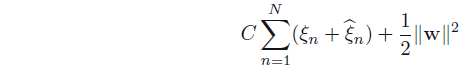ξn0$\xi_n\geq0$
ξn^0$\hat{\xi_n}\geq0$
tny(xn)ξnϵ$t_n-y(x_n)-\xi_n\leq\epsilon$
y(xn)tnξ^nϵ$y(x_n)-t_n-\hat{\xi}_n\leq\epsilon$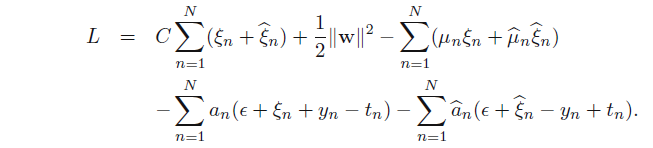## 3.对偶问题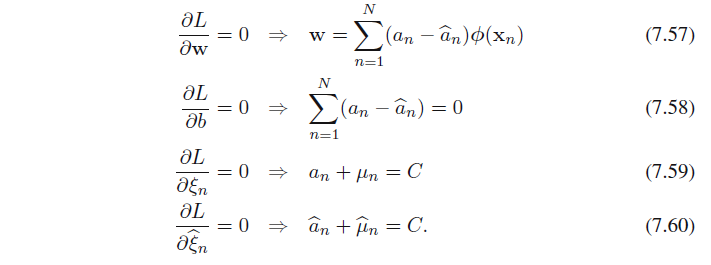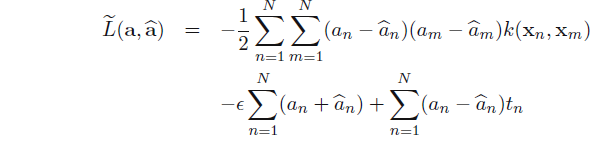0αnC$0\leq\alpha_n\leq C$
0αn^C$0\leq \hat{\alpha_n} \leq C$,其中k(xn,xm)=(xn)Txm$k(x_n,x_m)=(x_n)^Tx_m$为向量内积。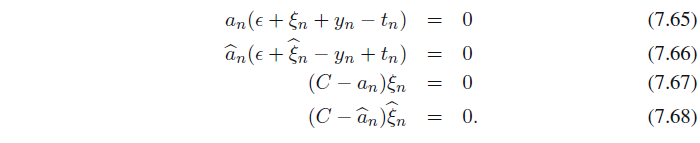αn0$\alpha_n\neq0$时，必有ϵ+ξn+y(xn)tn=0$\epsilon+\xi_n+y(x_n)-t_n=0$,这些点位于管道上方边界出，或者管道上面。
α^n0$\hat\alpha_n\neq0$时，必有ϵ+ξny(xn)+tn=0$\epsilon+\xi_n-y(x_n)+t_n=0$,这些点位于管道下方边界出，或者管道下面。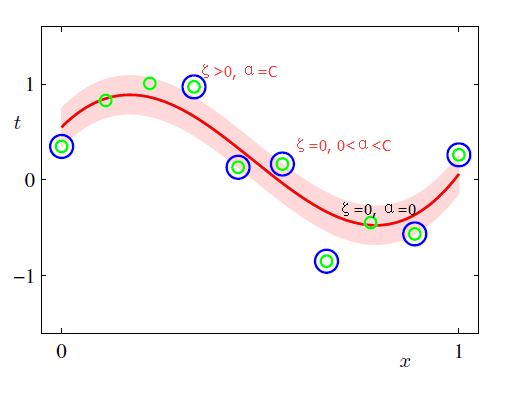## 4.超平面计算：

w$w$表达式带入到y=wTx+b$y=w^Tx+b$得：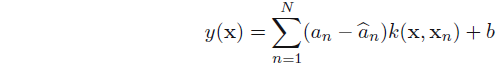ξn=0$\xi_n=0$
ϵ+ξn+y(xn)tn=0$\epsilon+\xi_n+y(x_n)-t_n=0$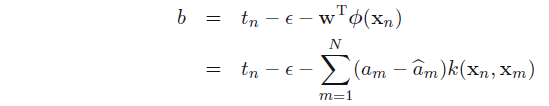#### 第一章：SVM

01-03

08-26749

#### 机器学习——SVM的回归形式(SVR)11-241万+

#### 支持向量机SVM和支持向量回归SVR简介——原理方法

07-181415

#### 如何使用支持向量机（Support Vector Machine，SVM）思想解决回归问题

04-08833

#### 一个运用SVM进行回归的例子

09-21262

#### SVM分类与回归

10-181万+

#### 机器学习中分类与回归问题的区别与联系

06-123735

#### 机器学习svm--正确率和召回率以及基于不平衡数据的分类调参

08-068462

#### 【机器学习】SVM之回归模型

02-25516

#### SVM-支持向量机（三）SVM回归与原理

09-042万+

#### 【机器学习】线性回归原理推导与算法描述

01-202万+

#### 机器学习中【回归算法】详解

06-022万+

#### 机器学习算法（一）SVM

07-1021万+

#### Java 最常见的 200+ 面试题：面试必备©️2020 CSDN 皮肤主题: 大白 设计师: CSDN官方博客点击重新获取扫码支付1.余额是钱包充值的虚拟货币，按照1:1的比例进行支付金额的抵扣。
2.余额无法直接购买下载，可以购买VIP、C币套餐、付费专栏及课程。余额充值